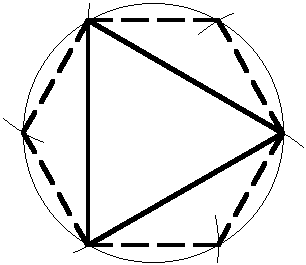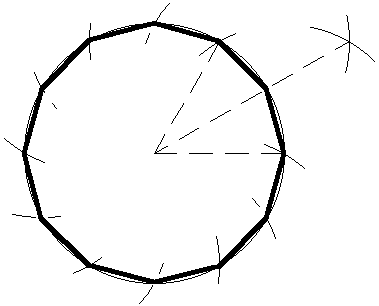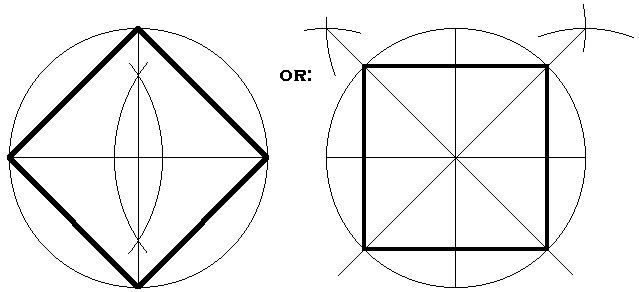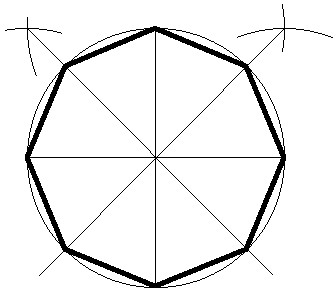INSCRIBING REGULAR POLYGONS

Part I

Polygons are closed plane figures whose edges are straight lines. Polygons are regular if all of their sides and angles are equal. This means that all the corners, or vertices, of a regular polygon will lie on a circle. Usually the simplest method, then, to construct a regular polygon is to inscribe it in a circle.

Inscribing an equilateral triangle and a hexagon

 Procedure: The radius of a circle can be struck exactly six times around the circle. Connecting the intersections of every other arc yields an equilateral triangle; connecting each successive intersection produces a six-sided figure or hexagon.Inscribing a dodecahedron

 Procedure: Set the compass to the radius of the circle and strike six equidistant arcs about its perimeter. Connect two neighboring intersections to the center of the circle. Bisect the resulting angle. Beginning at the intersection of the bisector and the circle strike six more arcs around the circle. There will be twelve equidistant intersections on the circle. These will mark the vertices of a dodecagon.Inscribing a squareProcedure: The tilted square, or diamond, was inscribed by connecting the ends of the horizontal and vertical diameters of a circle. A vertical diameter can be constructed as the perpendicular bisector of the horizontal diameter and vice-versa. The normal square was inscribed by connecting the diagonal diameters of the circle. These diameters were constructed by bisecting the right angles created by the horizontal and vertical diameters.

Inscribing an octagon

 Procedure: Construct horizontal and vertical diameters and then bisect the quadrants of the circle to divide it into eight segments. Connect the endpoints of the four diameters to create an octagon.The number of sides of any inscribed polygon may be doubled by further bisecting the segments of the circle. All of polygons above are doublings of the relatively simple constructions of the equilateral triangle and the square. Much more complex are the construction of figures like the pentagon (five sides). This is covered in part II.